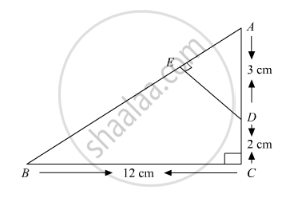Share

Books Shortlist
Your shortlist is empty

# Solution for In Below Figure, triangle Abc is Right Angled at C and De ⊥ Ab. Prove that δAbc ~ δAde and Hence Find the Lengths of Ae and De. - CBSE Class 10 - Mathematics

#### Question

In below Figure, ΔABC is right angled at C and DE ⊥ AB. Prove that ΔABC ~ ΔADE and Hence find the lengths of AE and DE.#### Solution

In ΔACB, by Pythagoras theorem

AB2 = AC2 + BC2

⇒ AB2 = (5)2 + (12)2

⇒ AB2 = 25 + 144 = 169

⇒ AB = sqrt169 = 13 cm

In ΔAED and ΔACB

∠A = ∠A [Common]

∠AED = ∠ACB [Each 90°]

Then, ΔAED ~ ΔACB [By AA similarity]

therefore"AE"/"AC"="DE"/"CB"="AD"/"AB"          [Corresponding parts of similar Δ are proportional]

rArr"AE"/5="DE"/12=3/13

rArr"AE"/5=3/13 and "DE"/12=3/13

"AE"=15/13 cm and "DE"=36/13 cm

Is there an error in this question or solution?

#### Video TutorialsVIEW ALL 

Solution for question: In Below Figure, triangle Abc is Right Angled at C and De ⊥ Ab. Prove that δAbc ~ δAde and Hence Find the Lengths of Ae and De. concept: Triangles Examples and Solutions. For the course CBSE
S